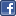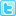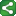Microsoft Excel Resource Center Free Macros, Formulas, Functions, Tutorials, Downloads, Add-Ins & More!
 Monte Carlo Tutorial power4XL Home
 Contents

Multi Coin Toss example VBA versionShareThis

Arrays can be used in various ways to manage data in Monte Carlo simulations.  Three of the basic uses are:

• Store only summarized results of data to be transferred to a worksheet

• Store data from each iteration as well as calculations performed within VBA on each iteration

• Store only the raw data from each iteration for dumping to a worksheet, then using the worksheet to perform further calculations and analyses

The earlier Simple Coin Toss 2 example was a rudimentary application of the first use identified above.  The Multi Coin Toss example that follows is a slightly more sophisticated way of using an array to capture and report summarized data - still without storing each iteration of data as a separate element in the array.  Later we'll provide examples of the latter two uses of arrays outlined above.

Now we'll apply the concepts of arrays and ranges to the VBA version of the Multi Coin Toss example we used earlier in the worksheet-only approach.  Here we'll also demonstrate writing the contents of an array to a spreadsheet range - the reverse of reading a range into an array.

To recap from our earlier example, in the Multi Coin Toss model we are tossing a coin ten times in each iteration, and recording how many times we get "heads" out of each ten tosses.  We can use this to calculate the probability of getting 5 heads out of ten tosses, or 7+ heads, etc.

Our worksheet is constructed as it appears below.  This is another example of the Hybrid approach, combining worksheet functions and VBA in the same model.

Note the Outputs section.  We use a VBA array to keep track of the number of 10-toss sets that result in the number of heads identified in the first column of the table.  We use worksheet functions to calculate the percentage in the third column.  The histogram below the output table is a simple Excel bar chart.

Now let's look at the VBA code behind the model.

Prev | Contents | Next

Copyright notice:  This site and all content, including computer code and spreadsheet examples, are copyright 2006 by Fritz Dooley.  License is granted for individual users to download examples and to copy code directly into user's spreadsheets and Visual Basic for Applications files.  Users may not redistribute code in any way.  Providing hyperlinks to this web site is encouraged, but posting code and examples on other web sites is expressly forbidden.  "Microsoft" is a registered trademark of Microsoft Corporation.   Neither this web site nor Fritz Dooley is affiliated with Microsoft Corporation.